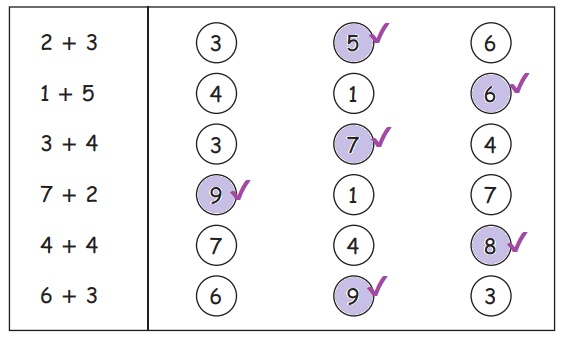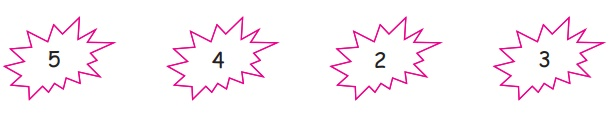Home | | Maths 1st Std | Addition

## Chapter: 1st Maths : Term 1 Unit 2 : Numbers

Keywords: Add, Total, Altogether, More, Combine together

Travel throughTeacher's note: Teacher can extend the story up to number 9.

Combine together

Learn

Addition means combining or putting things together

+ is the sign for addition.Practice

Count and Draw togetherPracticePractice4 + 3 =  7

3 + 4 =  7

5+3=8

3+5=8

3+2=5

2+3=5

Think like a mathematician2 + 1 and 1 + 2

Find all addition facts of 5.

1+4 = 5

2+3=5

3+2=5

4+1=5

Find all addition facts of 6.

1+5=6

2+4=6

3+3=6

4+2=6

5+1=6

Learn

5 + 1 = 6Practice2 + 3 = 5

4 + 5 = 9

1 + 7 = 8

4 + 2 = 6

6 +  3 = 9

3 + 3 = 6

3 + 4 = 7

7 + 2 = 9

Learn

3 + 5 = 8

3 + 5 = 8Practice2 + 3 = 5

4 + 4 = 8

5 + 1 = 6

2 + 7 = 9

2 + 3 = 5

4 + 4 = 8

5 + 1 = 6

2 + 7 = 9

Pleasure timeColour two numbers which make 8.Colour two numbers which make 9 in different ways.Try this

Circle the numbers which give a sum of 9.Mind math

Look at the first one and do the rest.Mind math (To be done orally)

1. Akilan has 4 pencils. Mukilan gives him 2 more. How many pencils does he have totally?4+2 =6

2. There are 5 f lowers in a plant and 3 flowers are there in another plant. How many flowers are there altogether?5+3= 8

3. Ruby's father gave her 6 chocolates and her mother gave 2 chocolates for her birthday. Totally how many chocolates does Ruby have in her hand?6+2=8

Think like a mathematician1. Pick a pair from the above numbers. Write addition fact for it.

3+2=9

2. Pick another pair of numbers. Write addition fact for it.

2+3=5

4+2=6

5+2=7

5+3=8

3. Keep doing it. How many different answers will you get?

9 different ways

5+4=9

5+2=7

5+3=8

4+5=9

4+2=6

4+3=7

2+5=7

2+4=6

2+3=5

Tags : Numbers | Term 1 Chapter 2 | 1st Maths , 1st Maths : Term 1 Unit 2 : Numbers
Study Material, Lecturing Notes, Assignment, Reference, Wiki description explanation, brief detail
1st Maths : Term 1 Unit 2 : Numbers : Addition | Numbers | Term 1 Chapter 2 | 1st Maths